# ML | Credit Card Fraud Detection

• Difficulty Level : Hard
• Last Updated : 05 Aug, 2022

The challenge is to recognize fraudulent credit card transactions so that the customers of credit card companies are not charged for items that they did not purchase.

Main challenges involved in credit card fraud detection are:

1. Enormous Data is processed every day and the model build must be fast enough to respond to the scam in time.
2. Imbalanced Data i.e most of the transactions (99.8%) are not fraudulent which makes it really hard for detecting the fraudulent ones
3. Data availability as the data is mostly private.
4. Misclassified Data can be another major issue, as not every fraudulent transaction is caught and reported.
5. Adaptive techniques used against the model by the scammers.

How to tackle these challenges?

1. The model used must be simple and fast enough to detect the anomaly and classify it as a fraudulent transaction as quickly as possible.
2. Imbalance can be dealt with by properly using some methods which we will talk about in the next paragraph
3. For protecting the privacy of the user the dimensionality of the data can be reduced.
4. A more trustworthy source must be taken which double-check the data, at least for training the model.
5. We can make the model simple and interpretable so that when the scammer adapts to it with just some tweaks we can have a new model up and running to deploy.

Before going to the code it is requested to work on a jupyter notebook. If not installed on your machine you can use Google colab.
Code : Importing all the necessary Libraries

 `# import the necessary packages``import` `numpy as np``import` `pandas as pd``import` `matplotlib.pyplot as plt``import` `seaborn as sns``from` `matplotlib ``import` `gridspec`

 `# Load the dataset from the csv file using pandas``# best way is to mount the drive on colab and ``# copy the path for the csv file``data ``=` `pd.read_csv(``"credit.csv"``)`

Code : Understanding the Data

 `# Grab a peek at the data``data.head()`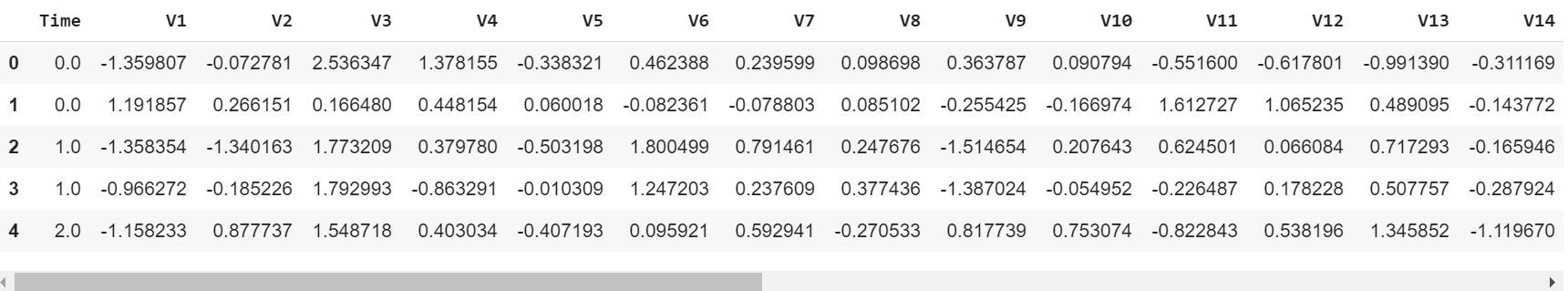Code : Describing the Data

 `# Print the shape of the data``# data = data.sample(frac = 0.1, random_state = 48)``print``(data.shape)``print``(data.describe())`

Output :

```(284807, 31)
Time            V1  ...         Amount          Class
count  284807.000000  2.848070e+05  ...  284807.000000  284807.000000
mean    94813.859575  3.919560e-15  ...      88.349619       0.001727
std     47488.145955  1.958696e+00  ...     250.120109       0.041527
min         0.000000 -5.640751e+01  ...       0.000000       0.000000
25%     54201.500000 -9.203734e-01  ...       5.600000       0.000000
50%     84692.000000  1.810880e-02  ...      22.000000       0.000000
75%    139320.500000  1.315642e+00  ...      77.165000       0.000000
max    172792.000000  2.454930e+00  ...   25691.160000       1.000000

[8 rows x 31 columns]

```

Code : Imbalance in the data
Time to explain the data we are dealing with.

 `# Determine number of fraud cases in dataset``fraud ``=` `data[data[``'Class'``] ``=``=` `1``]``valid ``=` `data[data[``'Class'``] ``=``=` `0``]``outlierFraction ``=` `len``(fraud)``/``float``(``len``(valid))``print``(outlierFraction)``print``(``'Fraud Cases: {}'``.``format``(``len``(data[data[``'Class'``] ``=``=` `1``])))``print``(``'Valid Transactions: {}'``.``format``(``len``(data[data[``'Class'``] ``=``=` `0``])))`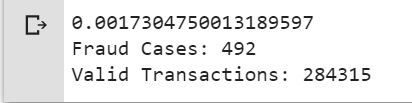Only 0.17% fraudulent transaction out all the transactions. The data is highly Unbalanced. Lets first apply our models without balancing it and if we don’t get a good accuracy then we can find a way to balance this dataset. But first, let’s implement the model without it and will balance the data only if needed.

Code : Print the amount details for Fraudulent Transaction

 `print``(“Amount details of the fraudulent transaction”)``fraud.Amount.describe()`

Output :

```Amount details of the fraudulent transaction
count     492.000000
mean      122.211321
std       256.683288
min         0.000000
25%         1.000000
50%         9.250000
75%       105.890000
max      2125.870000
Name: Amount, dtype: float64

```

Code : Print the amount details for Normal Transaction

 `print``(“details of valid transaction”)``valid.Amount.describe()`

Output :

```Amount details of valid transaction
count    284315.000000
mean         88.291022
std         250.105092
min           0.000000
25%           5.650000
50%          22.000000
75%          77.050000
max       25691.160000
Name: Amount, dtype: float64
```

As we can clearly notice from this, the average Money transaction for the fraudulent ones is more. This makes this problem crucial to deal with.

Code : Plotting the Correlation Matrix
The correlation matrix graphically gives us an idea of how features correlate with each other and can help us predict what are the features that are most relevant for the prediction.

 `# Correlation matrix``corrmat ``=` `data.corr()``fig ``=` `plt.figure(figsize ``=` `(``12``, ``9``))``sns.heatmap(corrmat, vmax ``=` `.``8``, square ``=` `True``)``plt.show()`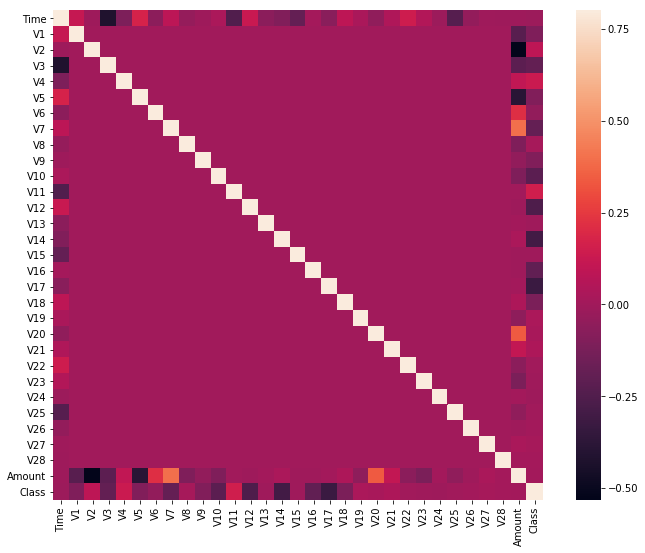In the HeatMap we can clearly see that most of the features do not correlate to other features but there are some features that either has a positive or a negative correlation with each other. For example, V2 and V5 are highly negatively correlated with the feature called Amount. We also see some correlation with V20 and Amount. This gives us a deeper understanding of the Data available to us.

Code : Separating the X and the Y values
Dividing the data into inputs parameters and outputs value format

 `# dividing the X and the Y from the dataset``X ``=` `data.drop([``'Class'``], axis ``=` `1``)``Y ``=` `data[``"Class"``]``print``(X.shape)``print``(Y.shape)``# getting just the values for the sake of processing ``# (its a numpy array with no columns)``xData ``=` `X.values``yData ``=` `Y.values`

Output :

```
(284807, 30)
(284807, )```

Training and Testing Data Bifurcation

We will be dividing the dataset into two main groups. One for training the model and the other for Testing our trained model’s performance.

 `# Using Scikit-learn to split data into training and testing sets``from` `sklearn.model_selection ``import` `train_test_split``# Split the data into training and testing sets``xTrain, xTest, yTrain, yTest ``=` `train_test_split(``        ``xData, yData, test_size ``=` `0.2``, random_state ``=` `42``)`

Code : Building a Random Forest Model using scikit learn

 `# Building the Random Forest Classifier (RANDOM FOREST)``from` `sklearn.ensemble ``import` `RandomForestClassifier``# random forest model creation``rfc ``=` `RandomForestClassifier()``rfc.fit(xTrain, yTrain)``# predictions``yPred ``=` `rfc.predict(xTest)`

Code : Building all kinds of evaluating parameters

 `# Evaluating the classifier``# printing every score of the classifier``# scoring in anything``from` `sklearn.metrics ``import` `classification_report, accuracy_score ``from` `sklearn.metrics ``import` `precision_score, recall_score``from` `sklearn.metrics ``import` `f1_score, matthews_corrcoef``from` `sklearn.metrics ``import` `confusion_matrix`` ` `n_outliers ``=` `len``(fraud)``n_errors ``=` `(yPred !``=` `yTest).``sum``()``print``(``"The model used is Random Forest classifier"``)`` ` `acc ``=` `accuracy_score(yTest, yPred)``print``(``"The accuracy is {}"``.``format``(acc))`` ` `prec ``=` `precision_score(yTest, yPred)``print``(``"The precision is {}"``.``format``(prec))`` ` `rec ``=` `recall_score(yTest, yPred)``print``(``"The recall is {}"``.``format``(rec))`` ` `f1 ``=` `f1_score(yTest, yPred)``print``(``"The F1-Score is {}"``.``format``(f1))`` ` `MCC ``=` `matthews_corrcoef(yTest, yPred)``print``(``"The Matthews correlation coefficient is{}"``.``format``(MCC))`

Output :

```The model used is Random Forest classifier
The accuracy is  0.9995611109160493
The precision is 0.9866666666666667
The recall is 0.7551020408163265
The F1-Score is 0.8554913294797689
The Matthews correlation coefficient is0.8629589216367891
```

Code : Visualizing the Confusion Matrix

 `# printing the confusion matrix``LABELS ``=` `[``'Normal'``, ``'Fraud'``]``conf_matrix ``=` `confusion_matrix(yTest, yPred)``plt.figure(figsize ``=``(``12``, ``12``))``sns.heatmap(conf_matrix, xticklabels ``=` `LABELS, ``            ``yticklabels ``=` `LABELS, annot ``=` `True``, fmt ``=``"d"``);``plt.title(``"Confusion matrix"``)``plt.ylabel(``'True class'``)``plt.xlabel(``'Predicted class'``)``plt.show()`

Output :

```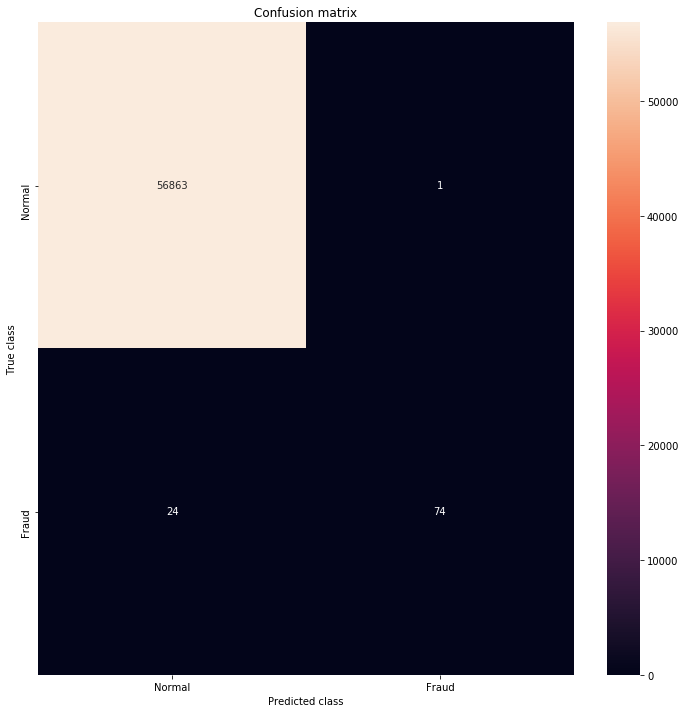```

Comparison with other algorithms without dealing with the imbalancing of the data.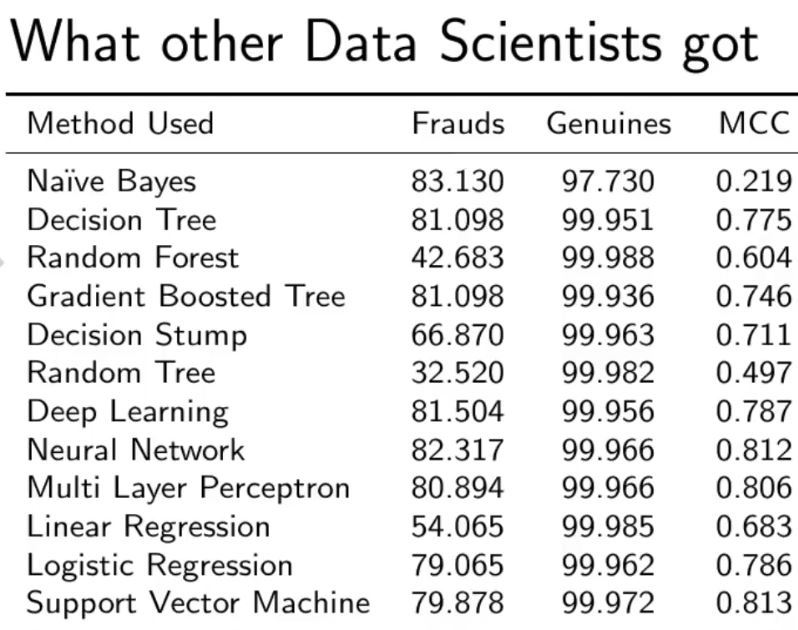As you can see with our Random Forest Model we are getting a better result even for the recall which is the most tricky part.

My Personal Notes arrow_drop_up